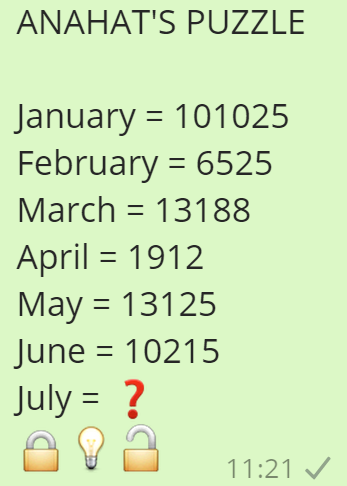# ANAHAT'S PUZZLE January = 101025 February = 6525 Then July = ?ANAHAT'S PUZZLE

January = 101025
February = 6525
March = 13188
April = 1912
May = 13125
June = 10215
July = ?

First letter count in the alphabet is first digit i.e. J > 10
Last letter count in the alphabet is first digit i.e. Y > 25

Now see this logic
January -- First Vowel is 'a' so middle digit is 1 (but repeated so 10)
February -- First Vowel is 'e' so middle digit is 5
March -- First Vowel is 'a' so middle digit is 1
April -- First Vowel is 'i' so middle digit is 9
May -- First Vowel is 'a' so middle digit is 1
June -- First Vowel is 'u' so middle digit is 21

July -- First vowel is u so middle digit is 21

Hence number for July is 102125